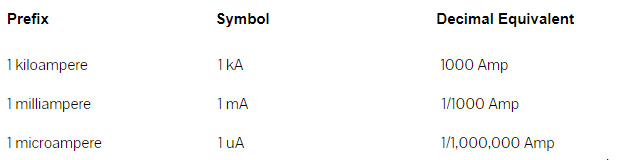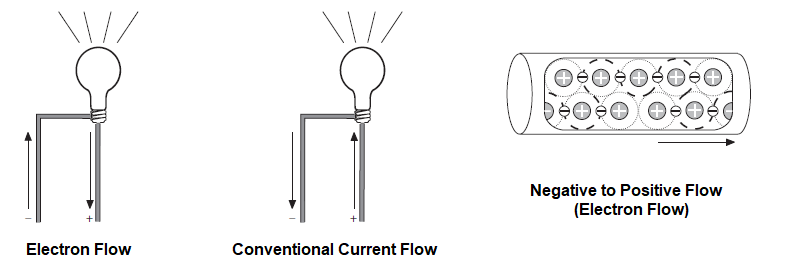## What is Current?

What is electric current?

Electricity is the flow of free electrons in a conductor from one atom to the next atom in the same general direction. This flow of electrons is referred to as current and is designated by the symbol “I”. Electrons move through a conductor at different rates and electric current has different values. Current is determined by the number of electrons that pass through a cross-section of a conductor in one second. We must remember that atoms are very small. It takes about 1,000,000,000,000,000,000,000,000 atoms to fill one cubic centimeter of a copper conductor. This number can be simplified using mathematical exponents. Instead of writing 24 zeros after the number 1, write 1024. Trying to measure even small values of current would result in unimaginably large numbers. For this reason current is measured in amperes, which is abbreviated “amps”. The letter “A” is the symbol for amps. A current of one amp means that in one second about 6.24 x 1018 electrons move through a cross-section of conductor. These numbers are given for information only and you do not need to be concerned with them. It is important, however, that the concept of current flow be understood.

Measuring Current

The following chart reflects special prefixes that are used when dealing with very small or large values of current:Direction of Current Flow

Some authorities distinguish between electron flow and current flow. Conventional current flow theory ignores the flow of electrons and states that current flows from positive to negative. To avoid confusion, we will use the electron flow concept which states that electrons flow from negative to positive.Posted in